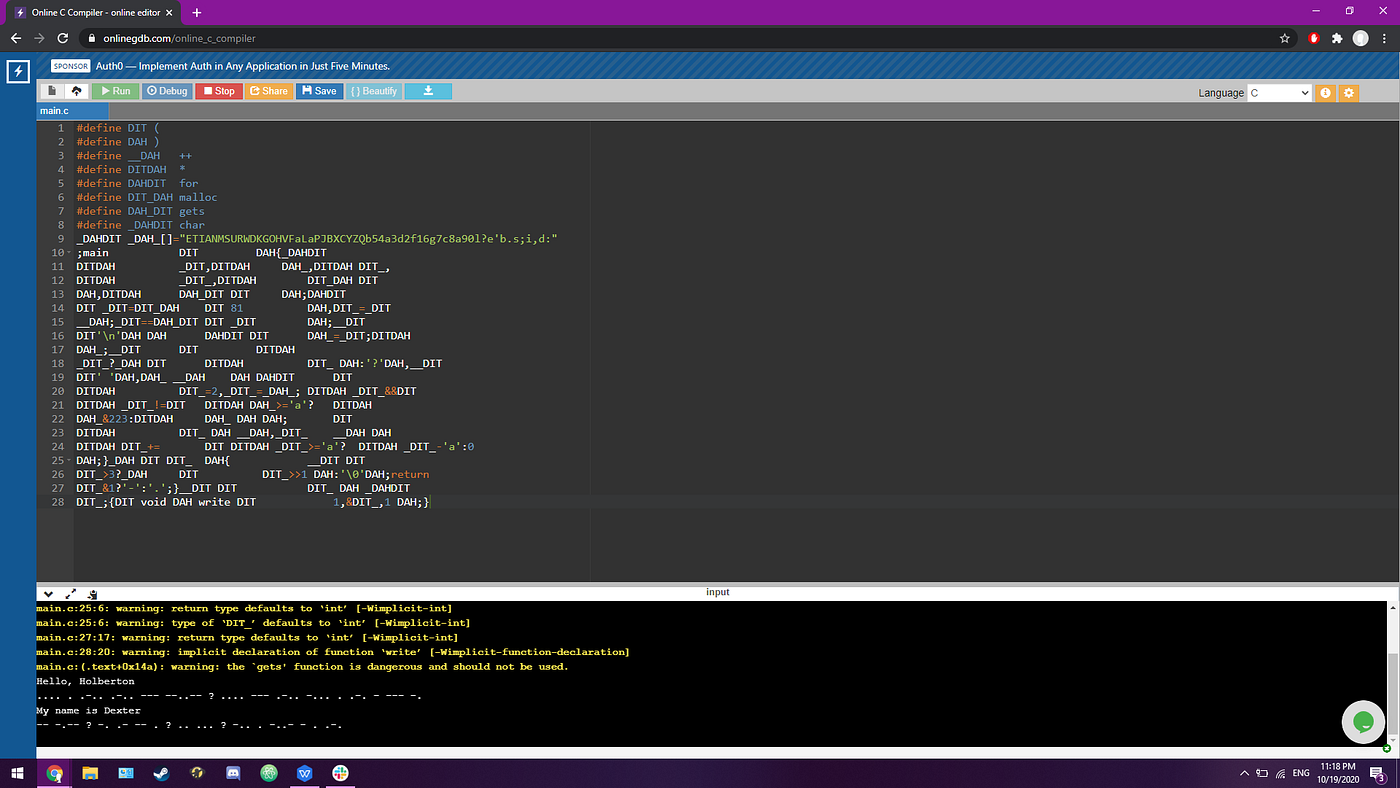# Hague: A Story of Macro expansions

`#define DIT (#define DAH )#define __DAH   ++#define DITDAH  *#define DAHDIT  for#define DIT_DAH malloc#define DAH_DIT gets#define _DAHDIT char_DAHDIT _DAH_[]="ETIANMSURWDKGOHVFaLaPJBXCYZQb54a3d2f16g7c8a90l?e'b.s;i,d:";main           DIT         DAH{_DAHDITDITDAH          _DIT,DITDAH     DAH_,DITDAH DIT_,DITDAH          _DIT_,DITDAH        DIT_DAH DITDAH,DITDAH      DAH_DIT DIT     DAH;DAHDITDIT _DIT=DIT_DAH    DIT 81          DAH,DIT_=_DIT__DAH;_DIT==DAH_DIT DIT _DIT        DAH;__DITDIT'\n'DAH DAH      DAHDIT DIT      DAH_=_DIT;DITDAHDAH_;__DIT      DIT         DITDAH_DIT_?_DAH DIT      DITDAH          DIT_ DAH:'?'DAH,__DITDIT' 'DAH,DAH_ __DAH    DAH DAHDIT      DITDITDAH          DIT_=2,_DIT_=_DAH_; DITDAH _DIT_&&DITDITDAH _DIT_!=DIT   DITDAH DAH_>='a'?   DITDAHDAH_&223:DITDAH     DAH_ DAH DAH;       DITDITDAH          DIT_ DAH __DAH,_DIT_    __DAH DAHDITDAH DIT_+=       DIT DITDAH _DIT_>='a'?  DITDAH _DIT_-'a':0DAH;}_DAH DIT DIT_  DAH{            __DIT DITDIT_>3?_DAH     DIT          DIT_>>1 DAH:'\0'DAH;returnDIT_&1?'-':'.';}__DIT DIT           DIT_ DAH _DAHDITDIT_;{DIT void DAH write DIT            1,&DIT_,1 DAH;}`
`main(){ Char *_(,*)_, * (_, * _(_,*malloc (), * gets ( ); for( _(=malloc(81),(_=_(++;_(==gets ( _( );__((‘\n’) )for( )_=_(;*)_;__(( *_(_?_)( *(_ ):’?’),__((‘ ‘),)_ ++) for(*(_=2,_(_=_DAH_; *_(_&&(* _(_!=( * )_>=’a’? *)_&223:* )_ ) );(*(_ ) ++,_(_++ )* (_+= ( * _(_>=’a’?*_(_-’a’:0);}_) ( (_ ){ __( ((_>3?_) ( (_>>1 ):’\0');return(_&1?’-’:’.’;}__( ( (_ ) char(_;{( void ) write (1,&(_,1 );}`
`#define MACRO data`
`gcc filename.c -E -o hague`
`# 1 "hague.c"# 1 "<built-in>"# 1 "<command-line>"# 31 "<command-line>"# 1 "/usr/include/stdc-predef.h" 1 3 4# 32 "<command-line>" 2# 1 "hague.c"# 9 "hague.c"char _DAH_[]="ETIANMSURWDKGOHVFaLaPJBXCYZQb54a3d2f16g7c8a90l?e'b.s;i,d:";main ( ){char* _DIT,* DAH_,* DIT_,* _DIT_,* malloc (),* gets ( );for( _DIT=malloc ( 81 ),DIT_=_DIT++;_DIT==gets ( _DIT );__DIT('\n') ) for ( DAH_=_DIT;*DAH_;__DIT ( *_DIT_?_DAH ( * DIT_ ):'?'),__DIT(' '),DAH_ ++ ) for (* DIT_=2,_DIT_=_DAH_; * _DIT_&&(* _DIT_!=( * DAH_>='a'? *DAH_&223:* DAH_ ) ); (* DIT_ ) ++,_DIT_ ++ )* DIT_+= ( * _DIT_>='a'? * _DIT_-'a':0);}_DAH ( DIT_ ){ __DIT (DIT_>3?_DAH ( DIT_>>1 ):'\0');returnDIT_&1?'-':'.';}__DIT ( DIT_ ) charDIT_;{( void ) write ( 1,&DIT_,1 );}`
`char _DAH_[]="ETIANMSURWDKGOHVFaLaPJBXCYZQb54a3d2f16g7c8a90l?e'b.s;i,d:";main ( ){Char *str1, *str2, *str3, *str4, *malloc ( ), *gets ( );for ( str1 = malloc( 81 ) , str3 = str1++; str1 == gets(str1); __DIT('\n') )for ( str2 = str1; *str2;  __DIT( *str4 ? _DAH ( *str3 ) : '?' ), __DIT(' '), str2++ )for (*str3 = 2, str4 = _DAH_; *str4 && (*str4 != ( *str2 >= 'a' ? *str2 & 223 : *str2 ) ); (*str3 )++, str4++ )*str3 += ( *str4 >= 'a' ? *str4 - 'a' : 0);}_DAH(str3){__DIT ( str3 > 3 ? _DAH ( str3>>1 ) : '\0');return str3 &1 ? '-' : '.' ;}__DIT (str3)char str3;{(void) write ( 1, &str3,1 );}`Example of code running with the original mess and example output

--

--